# ICSE Class 8 Maths Selina Solutions Chapter 10 Direct and Inverse Variations

In real life, we come across many such incidents where a change in one quantity leads to the change in another quantity. For example, if the speed of a car is increased then the time taken to cover the same distance will decrease. Let’s take one more example if we want the construction of a building to finish soon then we need to deploy more workers. So, the more the number of workers less will be time taken to complete the work. By now you must have understood that there is some relation between them as the variation in one quantity is bringing the variation in other quantity. You will learn all these concepts in chapter 10 of ICSE Class 8 Maths.

For students convenience, we have provided a detailed solution in pdf format for ICSE Class 8 Maths Selina Solutions Chapter 10 Direct and Inverse Variations. These Selina Solutions are prepared by the experienced teachers in the simplest form. By going through them students can easily get to know how the questions are being solved. Also, these solutions will help students to prepare for the annual exams. Click on the link below to download the Solution PDF.

## Download ICSE Class 8 Maths Selina Solutions PDF for Chapter 10:-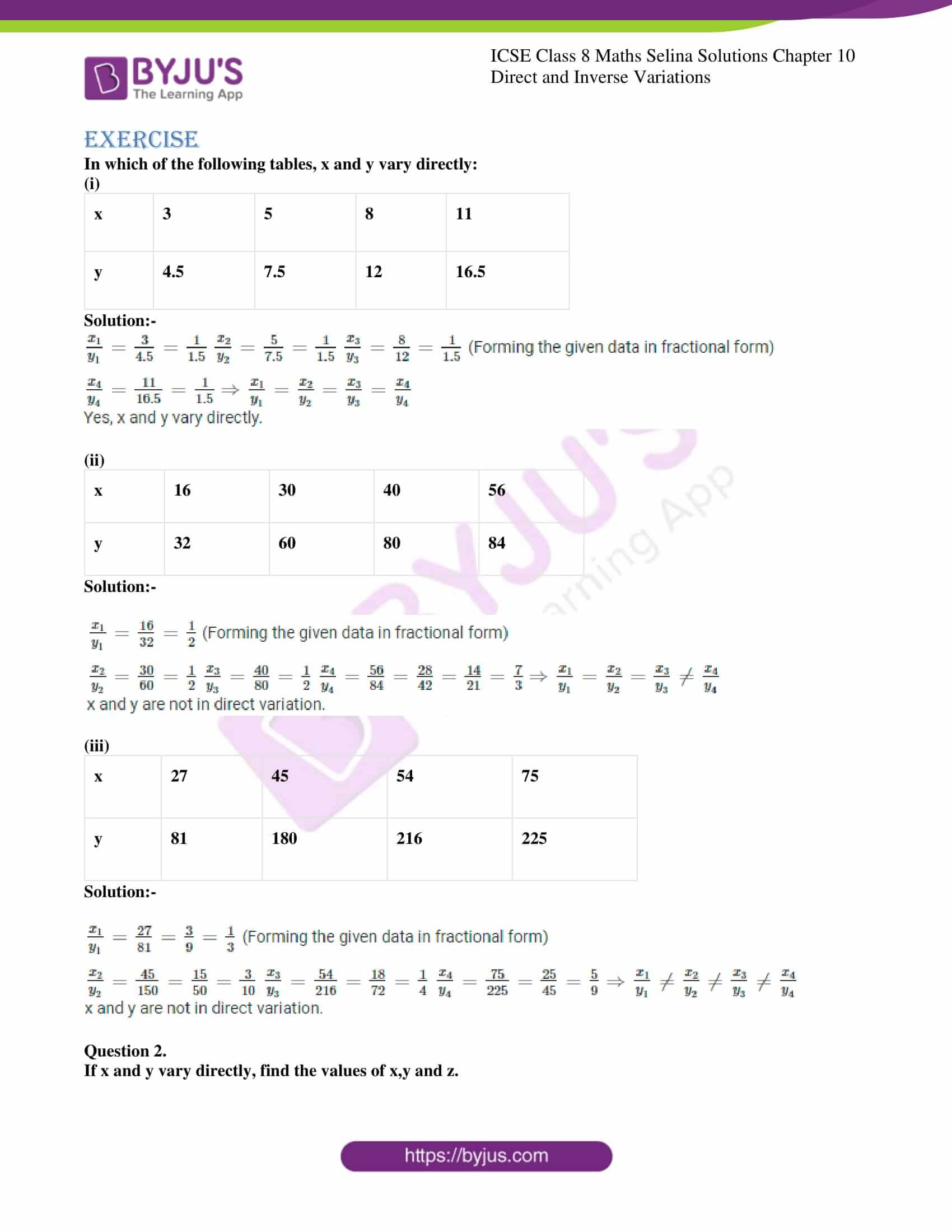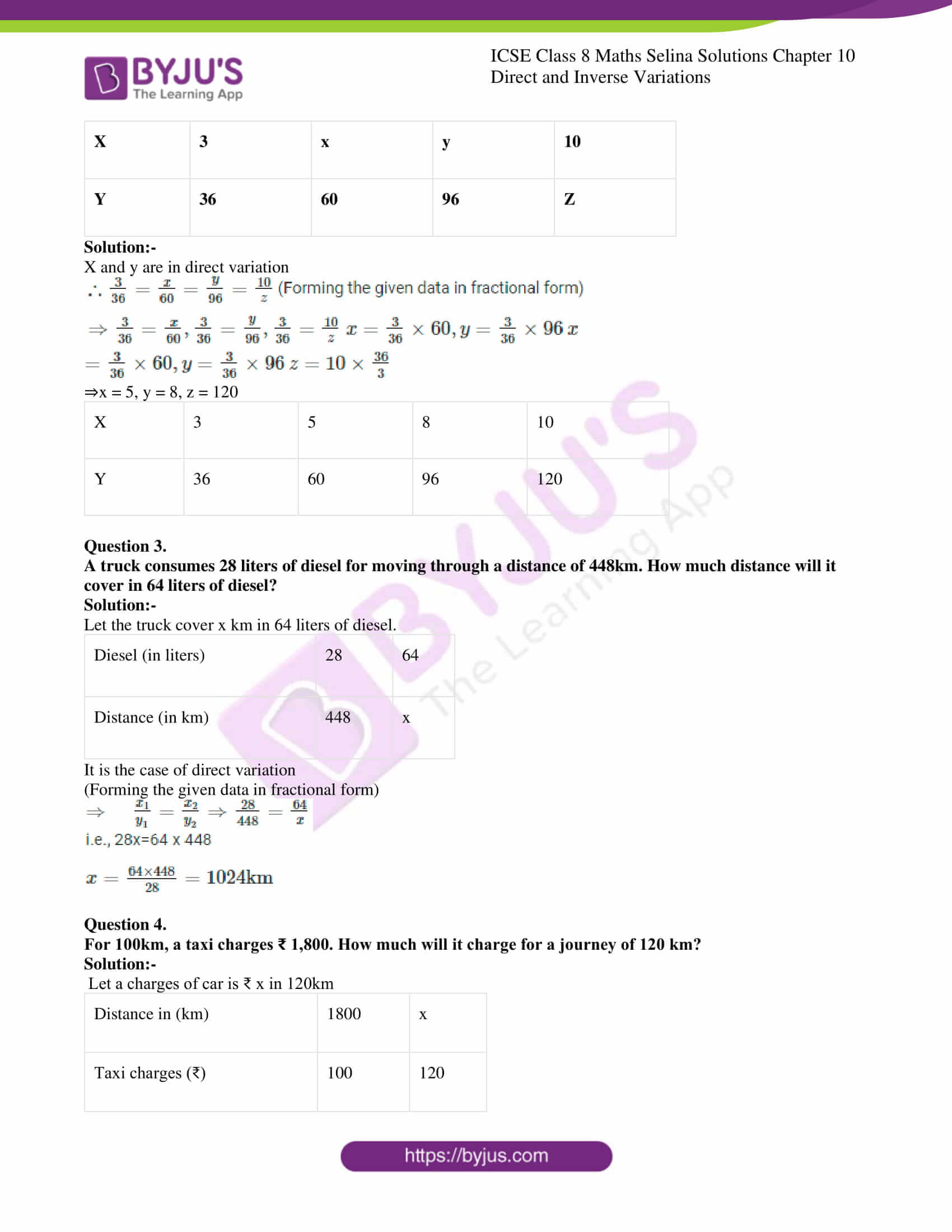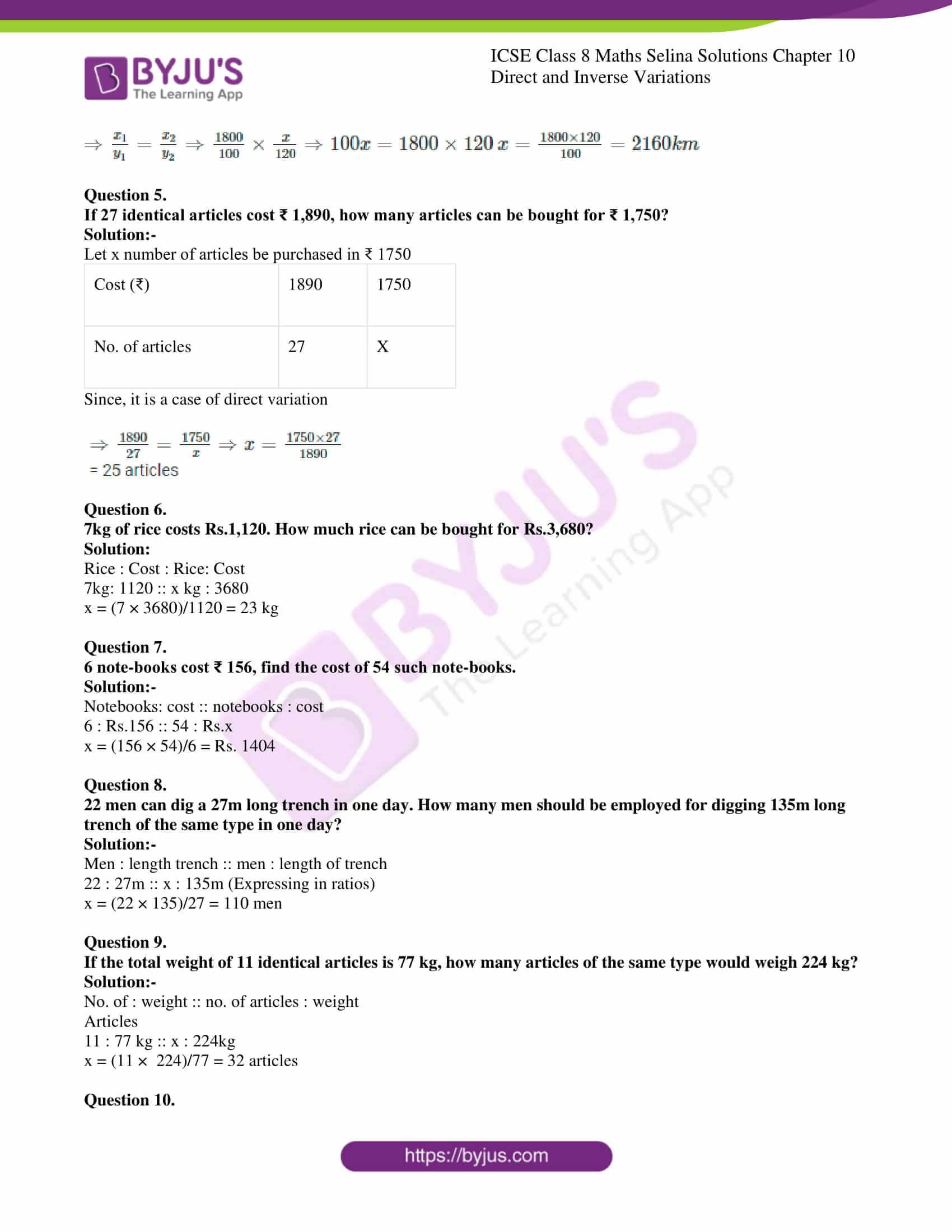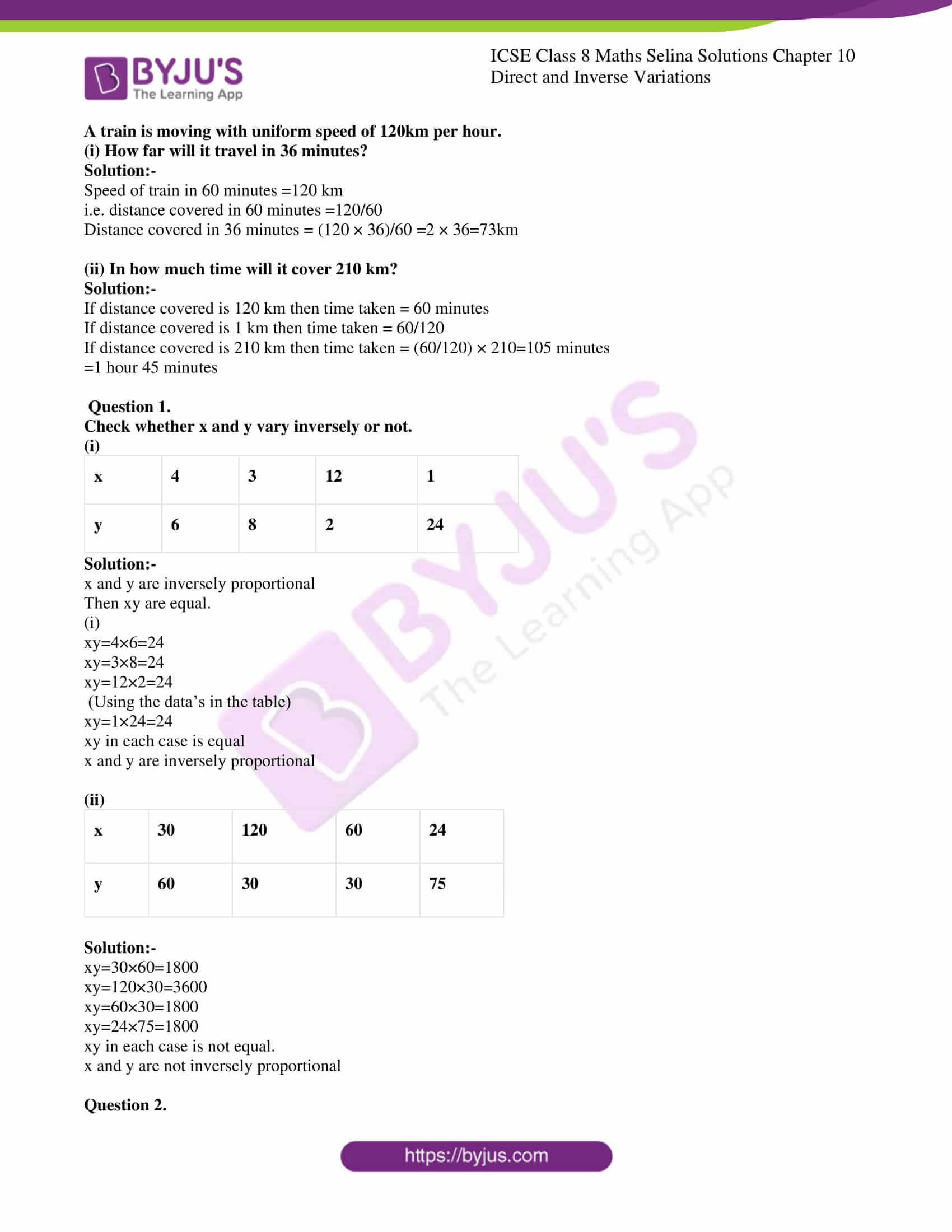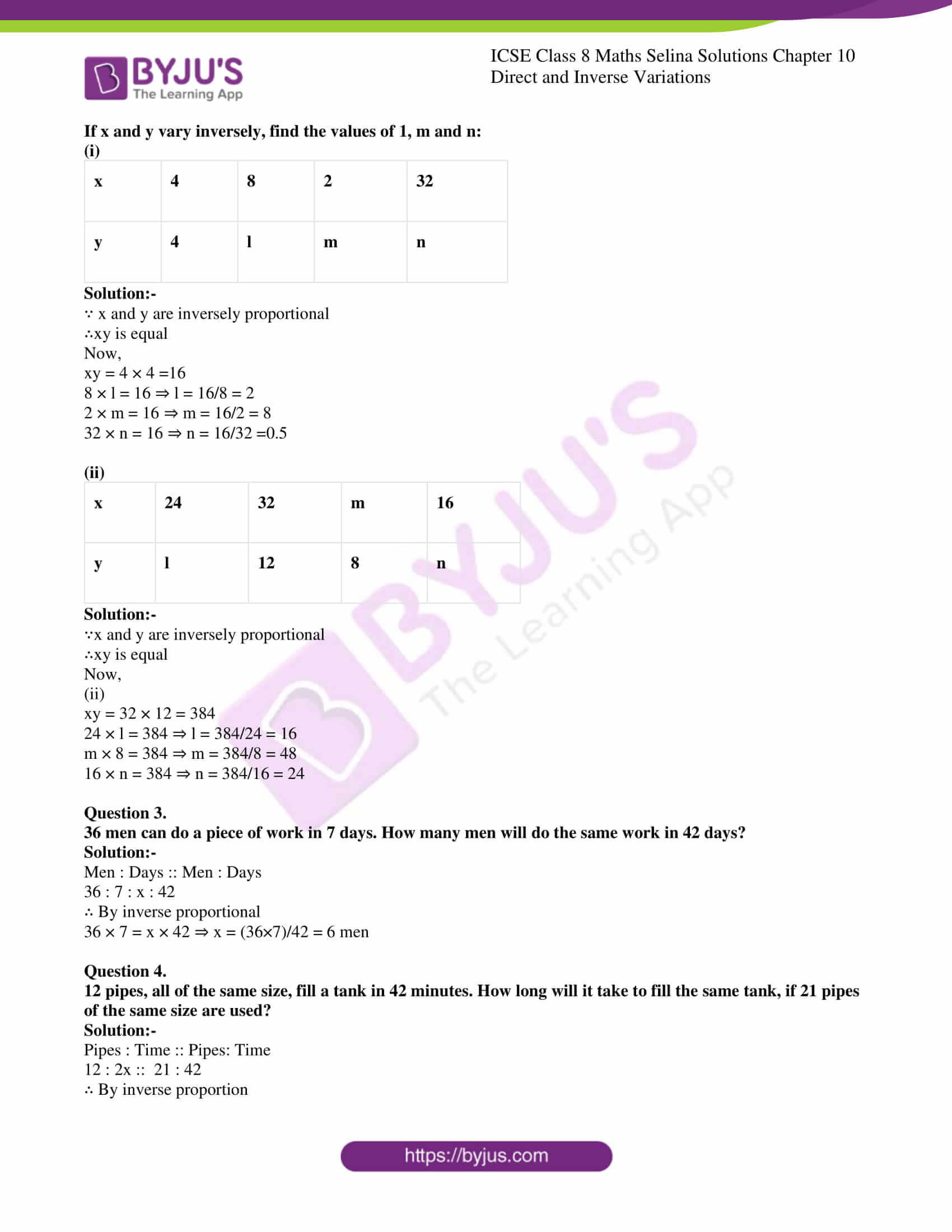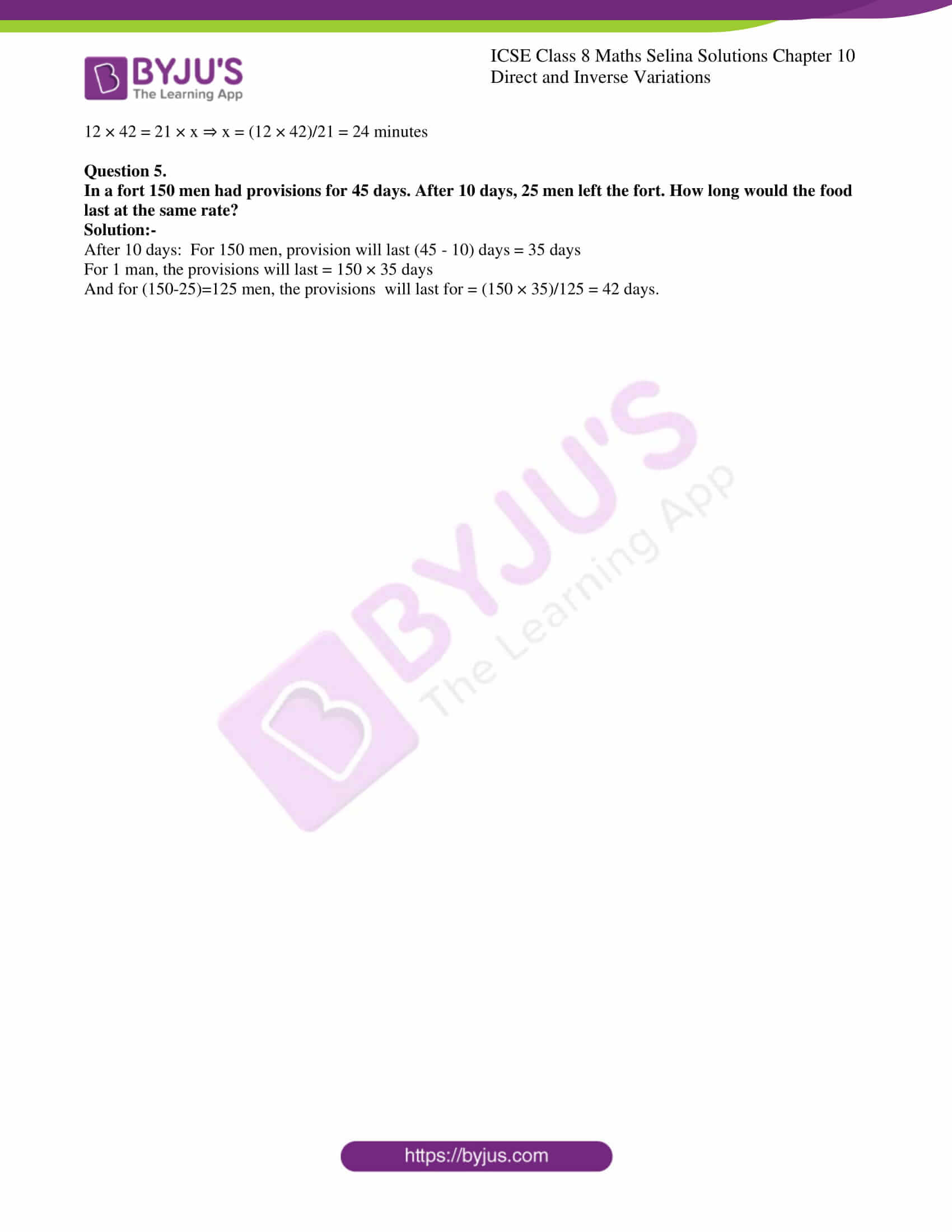ICSE Class 8 Maths Chapter 10 Direct and Inverse Variations has only 5 questions. The detailed solution of all these questions are provided below:

### ICSE Class 8 Maths Selina Solutions Chapter 10 – Direct and Inverse Variations

In which of the following tables, x and y vary directly:

(i)

 x 3 5 8 11 y 4.5 7.5 12 16.5

Solution:-

$$\begin{array}{l}\frac{x_{1}}{y_{1}}=\frac{3}{4.5}=\frac{1}{1.5}\end{array}$$
$$\begin{array}{l}\frac{x_{2}}{y_{2}}=\frac{5}{7.5}=\frac{1}{1.5}\end{array}$$
$$\begin{array}{l}\frac{x_{3}}{y_{3}}=\frac{8}{12}=\frac{1}{1.5} \end{array}$$
(Forming the given data in fractional form)

$$\begin{array}{l}\frac{x_{4}}{y_{4}}=\frac{11}{16.5}=\frac{1}{1.5}\end{array}$$
$$\begin{array}{l}\Rightarrow \frac{x_{1}}{y_{1}}=\frac{x_{2}}{y_{2}}=\frac{x_{3}}{y_{3}}=\frac{x_{4}}{y_{4}}\end{array}$$

Yes, x and y vary directly.

(ii)

 x 16 30 40 56 y 32 60 80 84

Solution:-

$$\begin{array}{l}\frac{x_{1}}{y_{1}}=\frac{16}{32}=\frac{1}{2}\end{array}$$
(Forming the given data in fractional form)

$$\begin{array}{l}\frac{x_{2}}{y_{2}}=\frac{30}{60}=\frac{1}{2}\end{array}$$
$$\begin{array}{l}\frac{x_{3}}{y_{3}}=\frac{40}{80}=\frac{1}{2}\end{array}$$
$$\begin{array}{l}\frac{x_{4}}{y_{4}}=\frac{56}{84}=\frac{28}{42}=\frac{14}{21}=\frac{7}{3}\end{array}$$
$$\begin{array}{l}\Rightarrow \frac{x_{1}}{y_{1}}=\frac{x_{2}}{y_{2}}=\frac{x_{3}}{y_{3}} \neq \frac{x_{4}}{y_{4}}\end{array}$$

x and y are not in direct variation.

(iii)

 x 27 45 54 75 y 81 180 216 225

Solution:-

$$\begin{array}{l}\frac{x_{1}}{y_{1}}=\frac{27}{81}=\frac{3}{9}=\frac{1}{3}\end{array}$$
(Forming the given data in fractional form)

$$\begin{array}{l}\frac{x_{2}}{y_{2}}=\frac{45}{150}=\frac{15}{50}=\frac{3}{10}\end{array}$$
$$\begin{array}{l}\frac{x_{3}}{y_{3}}=\frac{54}{216}=\frac{18}{72}=\frac{1}{4}\end{array}$$
$$\begin{array}{l}\frac{x_{4}}{y_{4}}=\frac{75}{225}=\frac{25}{45}=\frac{5}{9} \end{array}$$
$$\begin{array}{l}\Rightarrow \frac{x_{1}}{y_{1}} \neq \frac{x_{2}}{y_{2}} \neq \frac{x_{3}}{y_{3}} \neq \frac{x_{4}}{y_{4}}\end{array}$$

x and y are not in direct variation.

Question 2.

If x and y vary directly, find the values of x,y and z.

 X 3 x y 10 Y 36 60 96 Z

Solution:-

X and y are in direct variation

$$\begin{array}{l}∴ \frac{3}{36}=\frac{x}{60}=\frac{y}{96}=\frac{10}{z} \end{array}$$
(Forming the given data in fractional form)

$$\begin{array}{l}\Rightarrow \frac{3}{36}=\frac{x}{60}, \frac{3}{36}=\frac{y}{96}, \frac{3}{36}=\frac{10}{z}\end{array}$$
$$\begin{array}{l}x=\frac{3}{36} \times 60, y=\frac{3}{36} \times 96 \end{array}$$
$$\begin{array}{l}x=\frac{3}{36} \times 60, y=\frac{3}{36} \times 96\end{array}$$
$$\begin{array}{l}z=10 \times \frac{36}{3}\end{array}$$

⇒x=5,y=8,z=120

 X 3 5 8 10 Y 36 60 96 120

Question 3.

A truck consumes 28 liters of diesel for moving through a distance of 448km. How much distance will it cover in 64 liters of diesel?

Solution:-

Let the truck cover x km in 64 liters of diesel.

 Diesel (in liters) 28 64 Distance (in km) 448 x

It is the case of direct variation

(Forming the given data in fractional form)

$$\begin{array}{l}\Rightarrow \quad \frac{x_{1}}{y_{1}}=\frac{x_{2}}{y_{2}} \Rightarrow \frac{28}{448}=\frac{64}{x}\end{array}$$

i.e., 28x=64 x 448

$$\begin{array}{l}x=\frac{64 \times 448}{28}=1024 \mathrm{km}\end{array}$$

Question 4.

For 100km, a taxi charges ₹ 1,800. How much will it charge for a journey of 120 km?

Solution:-

Let a charges of car is ₹ x in 120km

 Distance in (km) 1800 x Taxi charges (₹) 100 120
$$\begin{array}{l}\Rightarrow \frac{x_{1}}{y_{1}}=\frac{x_{2}}{y_{2}} \Rightarrow \frac{1800}{100} \times \frac{x}{120}\end{array}$$
$$\begin{array}{l}\Rightarrow 100 x=1800 \times 120\end{array}$$
$$\begin{array}{l}x=\frac{1800 \times 120}{100}=2160 km\end{array}$$

Question 5.

If 27 identical articles cost ₹ 1,890, how many articles can be bought for ₹ 1,750?

Solution:-

Let x number of articles be purchased in ₹ 1750

 Cost (₹) 1890 1750 No. of articles 27 X

Since, it is a case of direct variation

$$\begin{array}{l}\Rightarrow \frac{1890}{27}=\frac{1750}{x}\end{array}$$
$$\begin{array}{l}\Rightarrow x=\frac{1750 \times 27}{1890}\end{array}$$

= 25 articles

Question 6.

7kg of rice costs Rs.1,120. How much rice can be bought for Rs.3,680?

Solution:

Rice : Cost : Rice: Cost

7kg: 1120 :: x kg : 3680

$$\begin{array}{l}∴ x=\frac{7 \times 3680}{1120}=23 \mathrm{kg}\end{array}$$

Question 7.

6 note-books cost ₹ 156, find the cost of 54 such note-books.

Solution:-

Notebooks: cost :: notebooks : cost

6 : Rs.156 :: 54 : Rs.x

$$\begin{array}{l}∴ x=\frac{156 \times 54}{6}=R s .1404\end{array}$$

Question 8.

22 men can dig a 27m long trench in one day. How many men should be employed for digging 135m long trench of the same type in one day?

Solution:-

Men : length trench :: men : length of trench

22 : 27m :: x : 135m (Expressing in ratios)

$$\begin{array}{l}x=\frac{22 \times 135}{27}=110 \mathrm{men}\end{array}$$

Question 9.

If the total weight of 11 identical articles is 77 kg, how many articles of the same type would weigh 224 kg?

Solution:-

No. of : weight :: no. of articles : weight

Articles

11 : 77 kg :: x : 224kg

$$\begin{array}{l}x=\frac{11 \times 224}{77}=32 articles\end{array}$$

Question 10.

A train is moving with uniform speed of 120km per hour.

(i) How far will it travel in 36 minutes?

Solution:-

Speed of train in 60 minutes =120 km

i.e. distance covered in 60 minutes

$$\begin{array}{l}=\frac{120}{60}\end{array}$$

Distance covered in 36 minutes

$$\begin{array}{l}=\frac{120 \times 36}{60} \end{array}$$
$$\begin{array}{l}=2 \times 36=73 \mathrm{km}\end{array}$$

(ii) In how much time will it cover 210 km?

Solution:-

If distance covered is 120 km then time taken = 60 minutes

If distance covered is 1 km then time taken

$$\begin{array}{l}=\frac{60}{120} \end{array}$$

If distance covered is 210 km then time taken

$$\begin{array}{l} =\frac{60}{120} \times 210=105 \end{array}$$
minutes

=1 hour 45 minutes

Question 1.

Check whether x and y vary inversely or not.

(i)

 x 4 3 12 1 y 6 8 2 24

Solution:-

x and y are inversely proportional

Then xy are equal.

(i)

$$\begin{array}{l}x y=4 \times 6=24\end{array}$$

$$\begin{array}{l}x y=3 \times 8=24\end{array}$$

$$\begin{array}{l}x y=12 \times 2=24\end{array}$$
(Using the data’s in the table)

$$\begin{array}{l}x y=1 \times 24=24\end{array}$$

xy in each case is equal

x and y are inversely proportional

(ii)

 x 30 120 60 24 y 60 30 30 75

Solution:-

$$\begin{array}{l}x y=30 \times 60=1800\end{array}$$

$$\begin{array}{l}x y=120 \times 30=3600\end{array}$$

$$\begin{array}{l}x y=60 \times 30=1800\end{array}$$

$$\begin{array}{l}x y=24 \times 75=1800\end{array}$$

xy in each case is not equal.

x and y are not inversely proportional

Question 2.

If x and y vary inversely, find the values of 1, m and n:

(i)

 x 4 8 2 32 y 4 l m n

Solution:-

∵ x and y are inversely proportional

∴xy is equal

Now,

$$\begin{array}{l}x y=4 \times 4=16\end{array}$$

$$\begin{array}{l}8 \times l=16 \Rightarrow l=\frac{16}{8}=2\end{array}$$

$$\begin{array}{l}2 \times m=16 \Rightarrow m=\frac{16}{2}=8\end{array}$$

$$\begin{array}{l}32 \times n=16 \Rightarrow n=\frac{16}{32}=0.5\end{array}$$

(ii)

 x 24 32 m 16 y l 12 8 n

Solution:-

∵x and y are inversely proportional

∴xy is equal

Now,

(ii)

$$\begin{array}{l}x y=32 \times 12=384\end{array}$$

$$\begin{array}{l}24 \times l=384 \Rightarrow l=\frac{384}{24}=16\end{array}$$

$$\begin{array}{l}m \times 8=384 \Rightarrow m=\frac{384}{8}=48\end{array}$$

$$\begin{array}{l}16 \times n=384 \Rightarrow n=\frac{384}{16}=24\end{array}$$

Question 3.

36 men can do a piece of work in 7 days. How many men will do the same work in 42 days?

Solution:-

Men : Days :: Men : Days

36 : 7 : x : 42

∴ By inverse proportional

$$\begin{array}{l}36 \times 7=x \times 42\end{array}$$
$$\begin{array}{l}\Rightarrow x=\frac{36 \times 7}{42}=6 \mathrm{men}\end{array}$$

Question 4.

12 pipes, all of the same size, fill a tank in 42 minutes. How long will it take to fill the same tank, if 21 pipes of the same size are used?

Solution:-

Pipes : Time :: Pipes: Time

12 : 2x ::  21 : 42

∴ By inverse proportion

$$\begin{array}{l}12 \times 42=21 \times x\end{array}$$
$$\begin{array}{l}\Rightarrow x=\frac{12 \times 42}{21}=24 minutes \end{array}$$

Question 5.

In a fort 150 men had provisions for 45 days. After 10 days, 25 men left the fort. How long would the food last at the same rate?

Solution:-

After 10 days:  For 150 men, provision will last (45-10) days =35 days

For 1 man, the provisions will last

$$\begin{array}{l}=150 \times 35 days \end{array}$$

And for (150-25)=125 men, the provisions  will last for

$$\begin{array}{l}=\frac{150 \times 35}{125}=42 \end{array}$$
days.

To get the Selina Solutions for all the chapters of class 8 Maths, visit the ICSE Class 8 Maths Selina Solutions page.

### ICSE Class 8 Maths Selina Solutions Chapter 10 – Direct and Inverse Variations

The application of direct and indirect variation can be seen in daily life. Mostly this concept is used in solving the problems related to:

• Time, speed and distance
• Time and work
• Pipe and cistern

Solving these questions will also improve your quantitative aptitude. So, you must practice all the questions provided in the chapter. In case you couldn’t able to solve any problem then refer to the solutions provided in the PDF. Moreover, you can also access the answers for all the exercises of Maths, Physics, Chemistry and Biology subjects by clicking on ICSE Class 8 Selina Solutions.

We hope this information on “ICSE Class 8 Maths Selina Solutions Chapter 10 Direct and Inverse Variations” is useful for students. Keep learning and stay tuned for further updates on ICSE and other competitive exams. To access interactive Maths and Science Videos download BYJU’S App and subscribe to YouTube Channel.